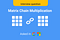# Matrix chain multiplication using Dynamic Programming

Matrix chain multiplication is an optimization problem concerning the most efficient way to multiply a given sequence of matricesThis would be helpful in cracking interviews of many elite companies!

# Introduction

This is going to be an in-depth explanation of matrix chain multiplication. This may be solved using brute force or overlapping subproblems but in this blog, we would be using the interesting principles of dynamic programming to explain it. I’ll be using handwritten notes compiled on OneNote by Microsoft to better explain the concepts in a comprehensible manner. I have also uploaded a video on YouTube on the same topic.(https://youtu.be/sgoqQzf3MqA)

# Problem statement

We need to find the most efficient way to multiply a…